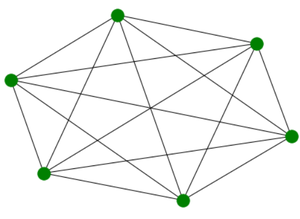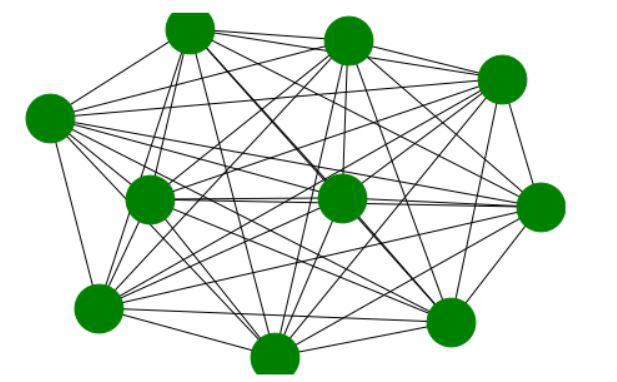Open In App

# Complete Graph using Networkx in Python

A complete graph also called a Full Graph it is a graph that has n vertices where the degree of each vertex is n-1. In other words, each vertex is connected with every other vertex.

Example: Complete Graph with 6 edges:C_G6

Properties of Complete Graph:

• The degree of each vertex is n-1.
• The total number of edges is n(n-1)/2.
• All possible edges in a simple graph exist in a complete graph.
• It is a cyclic graph.
• The maximum distance between any pair of nodes is 1.
• The chromatic number is n as every node is connected to every other node.
• Its complement is an empty graph.

We will use the networkx module for realizing a Complete graph. It comes with an inbuilt function networkx.complete_graph() and can be illustrated using the networkx.draw() method. This module in Python is used for visualizing and analyzing different kinds of graphs.

Syntax: networkx.complete_graph(n)

Parameters:

• N: Number of nodes in complete graph.
• Returns an networkx graph complete object.
• Nodes are indexed from zero to n-1.

Used to realize the graph by passing graph object.

networkx.draw(G, node_size, node_color)

Parameters:

• G: It refers to the complete graph object
• node_size: It refers to the size of nodes.
• node_color: It refers to color of the nodes.

Approach:

• We will import the required module networkx.
• Then we will create a graph object using networkx.complete_graph(n).
• Where n specifies n number of nodes.
• For realizing graph, we will use networkx.draw(G, node_color = ’green’, node_size=1500)
• The node_color and node_size arguments specify the color and size of graph nodes.

Example 1:

## Python3

 `# import required module``import` `networkx` `# create object``G ``=` `networkx.complete_graph(``6``)` `# illustrate graph``networkx.draw(G, node_color ``=` `'green'``,``              ``node_size ``=` `1500``)`

Output:Output

The output of the above program gives a complete graph with 6 nodes as output as we passed 6 as an argument to the complete_graph function.

Example 2:

## Python3

 `# import required module``import` `networkx` `# create object``G ``=` `networkx.complete_graph(``10``)` `# illustrate graph``networkx.draw(G, node_color ``=` `'green'``,``              ``node_size ``=` `1500``)`

Output:Advantages of using a complete graph in social network analysis include:

• Simplicity: Complete graphs are a simple structure that can be easily understood, making it easy to extract insights from the data.
• High connectivity: All nodes in a complete graph are connected to each other, which makes it easy to model all possible interactions between the nodes.
• Representing fully connected groups: Complete graphs can be used to represent groups where all members are fully connected, such as small teams or communities.

Disadvantages of using a complete graph in social network analysis include:

Limited representation of real-world networks: Complete graphs are a highly simplified representation of real-world networks, which may not accurately reflect the complexity and diversity of the relationships in a network.

High computational cost: Complete graphs have a high number of edges, which can make it computationally expensive to analyze and visualize.

Limited scalability: Complete graphs are not suitable for very large networks as the number of edges increases exponentially with the number of nodes.

### Reference:

“Python NetworkX: A Practical Overview” by Shai Vaingast is a good reference book for learning NetworkX and its application in social network analysis. The book covers the basics of NetworkX and its use in solving real-world problems such as community detection, centrality measures, and graph visualization. It also includes examples of creating and analyzing complete graphs using NetworkX.